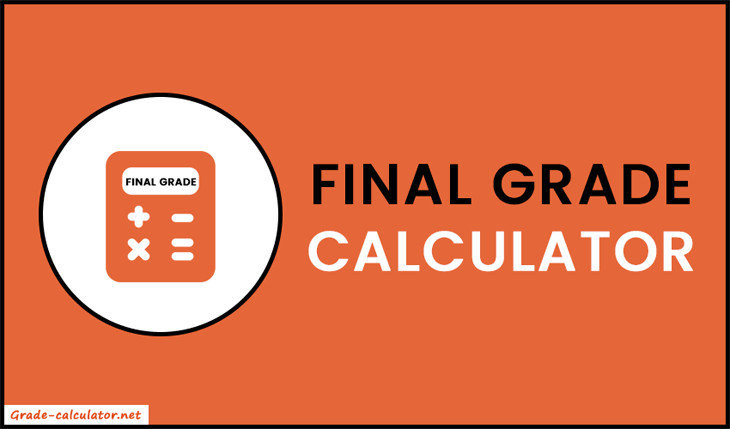%
%
%
%

## What is Final Grade Calculator?

A final grade calculator is a free educational tool that helps to find what grade a student needs to achieve on their final exam in order to reach a desired grade in a class. The calculator takes the student's current grade, desired grade, and weight of the final exam or assignment. By inputting these values into the calculator, the student can determine what grade they need to achieve on the final in order to reach their goal.

Our tool can be useful for students who are looking to improve their overall grades or maintain a certain level of academic achievement. It can also help students to set realistic goals and create a plan for achieving those goals.### How to Calculate Final Exam Grade?

To calculate the final grade, we will use the following formula:

 Desired - (100% - Final Weight) × Current Final Weight

In the above formula, the final exam weight should be in decimal form. To convert it into decimal, divide it by 100.

Here's an example:

Suppose the current grade is 74%, the required grade is 85%, and the final exam weight is 52%. Calculate the final grade.

Place above all values in the formula.

Final Grade = (85% - (100% - 52%) × 74%) / 52%

= (85 - ((100 - 52)/100) × 74) / (52/100)

= (85 - (48/100) × 74) / 0.52

= (85 - (0.48 × 74)) / 0.52

= (85 - 35.52) / 0.52

= 49.48 / 0.52

= 95.15%

Therefore, the student needs to score 95.15% in the final exam to reach the target grade of 85%. Our final grade calculator follows the same procedure and gives answers within a second. So, keep it handy or bookmark it to make complex calculations easier, faster, and more accurate.

### How to use Final Grade Calculator?

1. Firstly, input the current grade, desired grade, and weight of the final exam in the appropriate field.
2. Now press the "Calculate" button and our calculator starts the calculation in the backend and shows the final exam grade needed on your screen instantly.
3. To restart the process, press the "Reset" button.